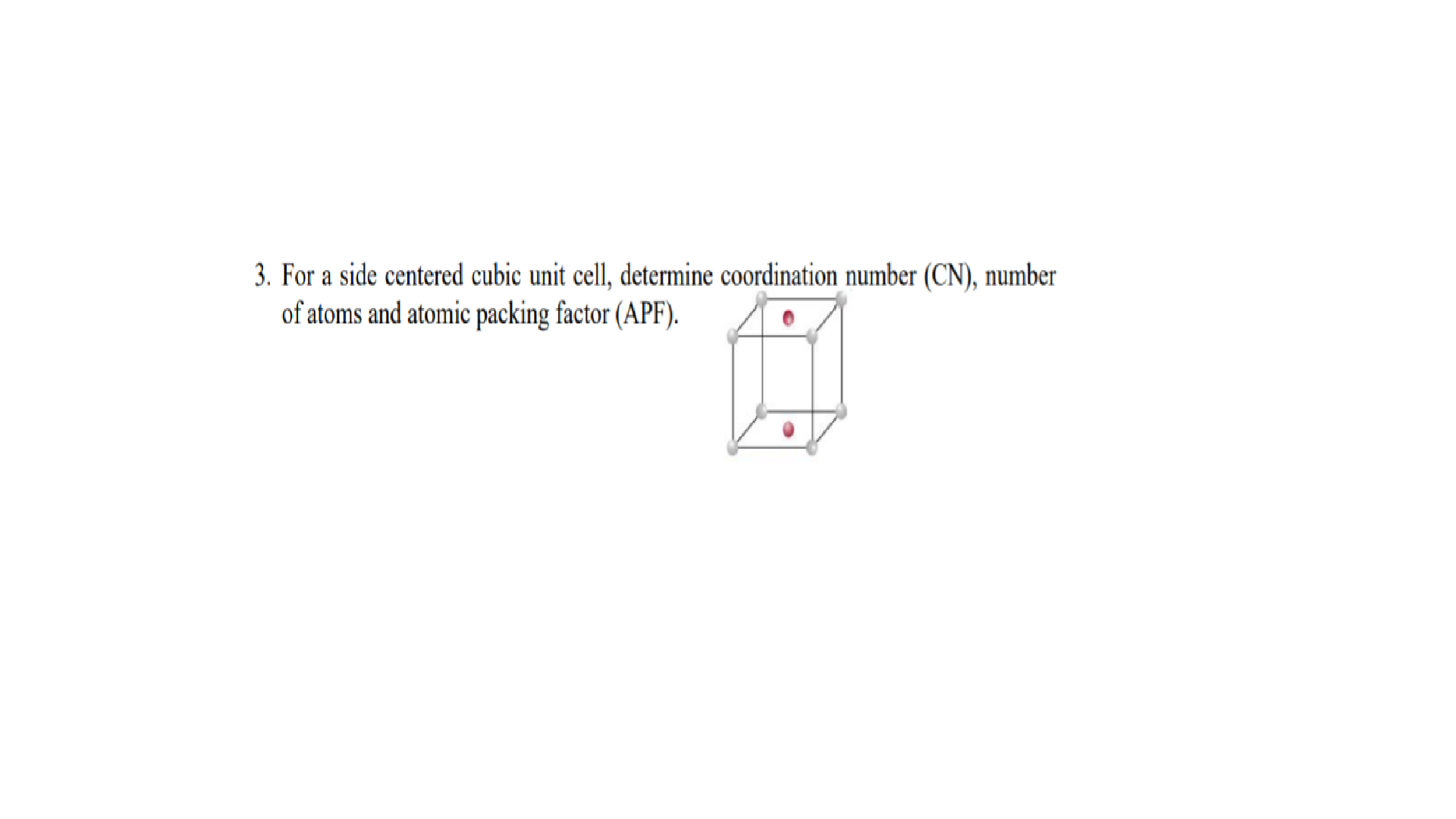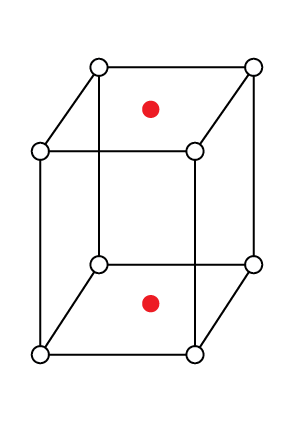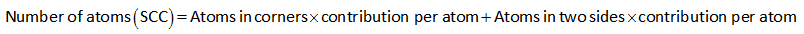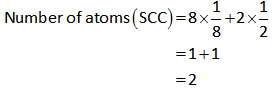# 3. For a side centered cubic unit cell, determine coordination number (CN), numberof atoms and atomic packing factor (APF).

Question
66 views

For a side centered cubic unit cell, determine coordination number (CN), number of atoms and atomic packing factor (APF).help_outlineImage Transcriptionclose3. For a side centered cubic unit cell, determine coordination number (CN), number of atoms and atomic packing factor (APF). fullscreen
check_circle

Introduction

Side centered cubic (SCC) is the crystal structure which possesses eight atoms on the eight corners and one atom exists on two parallel opposite faces. In SCC unit cell, the "number of atoms" (Z) is 2. SCC unit cell also termed as "end-centered cubic cell"

Calculation

The given side centered cubic unit cell is shown below.In the given centered cubic, 1 atom is surrounded by 6 adjacent atoms. For example, one corner atom is surrounded by  6 adjacent corner atoms.

Hence the coordination number of side centered cubic unit cell (SCC) is 6.

Calculation

The number of atoms (Z) present in the side centered cubic unit cell can be calculated as shown below.The contribution of corners in a unit cell is 1/8.

The contribution of ends (faces) in a unit cell is 1/2.

The total number of atoms in corners is 8 and the total number of atoms in two sides is 2.

Substitute the values in the above formula.Hence the number of atoms (Z) in the side centered cubic unit cell is 2.

...

### Want to see the full answer?

See Solution

#### Want to see this answer and more?

Solutions are written by subject experts who are available 24/7. Questions are typically answered within 1 hour.*

See Solution
*Response times may vary by subject and question.
Tagged in